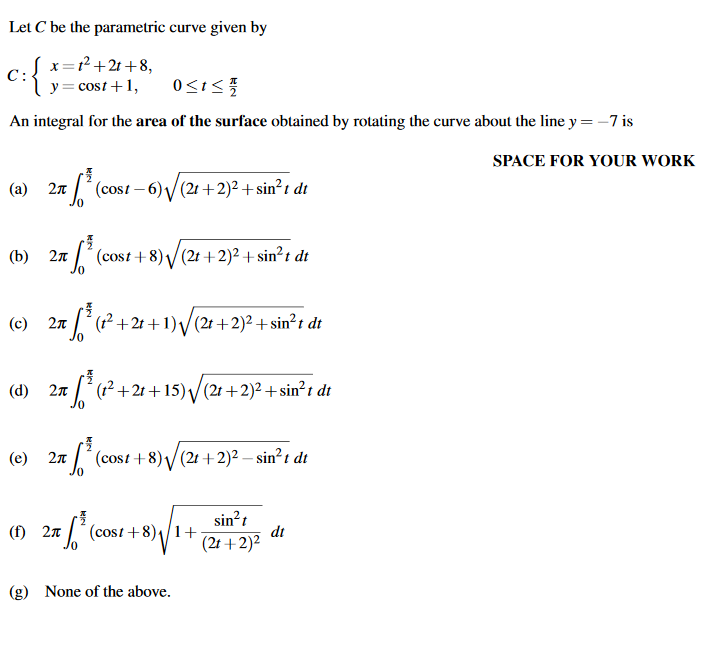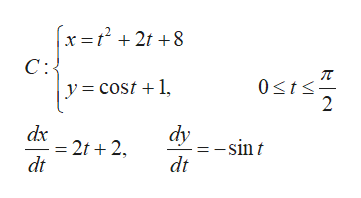# Let C be the parametric curve given by1²+2t +8,C:y= cost +1,An integral for the area of the surface obtained by rotating the curve about the line y =-7 isSPACE FOR YOUR WORK7´ (cost – 6) / (21 + 2)² + sin²t dt(a)2лt[´ (cost +8) /(2t +2)² + sin²t dt(b) 2л(1² +2t + 1)/(21 +2)2 + sin²t dt(c)(? +21+15) V(21 +2)² + sin²t dt(d)2лL (cost+8)V(21 +2)² – sin²t dt(e)sin?t1+(2t +2)²(cost +8)dt() 2л(g)None of the above.

Question
2 viewshelp_outlineImage TranscriptioncloseLet C be the parametric curve given by 1²+2t +8, C: y= cost +1, An integral for the area of the surface obtained by rotating the curve about the line y = -7 is SPACE FOR YOUR WORK 7´ (cost – 6) / (21 + 2)² + sin²t dt (a) 2л t[´ (cost +8) /(2t +2)² + sin²t dt (b) 2л (1² +2t + 1)/(21 +2)2 + sin²t dt (c) (? +21+15) V(21 +2)² + sin²t dt (d) 2л L (cost+8)V(21 +2)² – sin²t dt (e) sin?t 1+ (2t +2)² (cost +8) dt () 2л (g) None of the above. fullscreen
check_circle

Step 1

Given parametric curve ...help_outlineImage Transcriptionclosex =t + 2t +8 C:{ y = cost +1, Osts: dx = 2t + 2, dt dy = -sin t dt fullscreen

### Want to see the full answer?

See Solution

#### Want to see this answer and more?

Solutions are written by subject experts who are available 24/7. Questions are typically answered within 1 hour.*

See Solution
*Response times may vary by subject and question.
Tagged in

### Calculus